# SECTION 2: Geometrical relationships.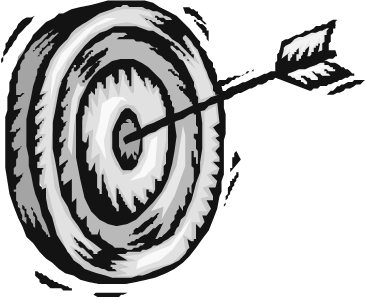### Specific Outcome

Explore, analyse and critique, describe and represent, interpret and justify geometrical relationships and conjectures.### Assessment Criteria

• Descriptions are based on a systematic analysis of the shapes and reflect the properties of the shapes accurately, clearly and completely. (SO 2, AC1)
• Descriptions include quantitative information appropriate to the situation and need. (SO 2, AC2)
• Conjectures as appropriate to the situation, are based on well-planned investigations of geometrical properties(SO 2, AC3)
• Representations of the problems are consistent with and appropriate to the problem context. The problems are represented comprehensively and in mathematical terms (SO 2, AC4).
• Results are achieved through efficient and correct analysis and manipulation of representations. (SO2, AC5)
• Problem-solving methods are presented clearly, logically and in mathematical terms. (SO 2, AC6)
• Solutions are correct and are interpreted and valid in terms of the context of the problem.(SO 2, AC7)

### 2. INTRODUCTION

Geometry is a branch of mathematics that studies spatial relationships and forms as well as relationships and forms that resemble spatial ones in structure.

The origin of the term “geometry,” which literally means “earth measurement,” may be explained in the following words, attributed to the ancient Greek scholar Eudemos of Rhodes (fourth century B.C.):“Geometry was discovered by the Egyptians as a result of their measurement of land. This measurement was indispensable to them because of the overflowing of the river Nile, which continually washed out boundaries.” The ancient Greeks already considered geometry to be a mathematical science, while for the science of measuring land the term “geodesy” was introduced. Judging by fragments that have been preserved from ancient Egyptian writings, one can see that geometry developed not only from measurements of land but also from measurements of volumes and surfaces in excavation and construction work.

### 2.1 PROPERTIES OF GEOMETRIC SHAPES

Each geometric shape has its own characteristics by which you can identify and recognize Geometry is the study of how lines interact with each other to form angles and shapes. It is necessary to appreciate the logical reasoning that goes with understanding the concepts of geometry so that its principles may be applied in various situations. We start with definitions and axioms (fundamental principles) as our foundation, and build up to the more complex theorems step-by-step.

#### 2.1.1 Polygons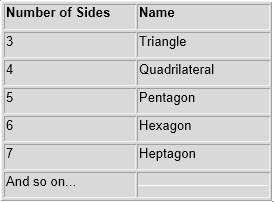A polygon is a closed figure composed of three or more line segments that intersect at their endpoints. Each line segment is called the side. The intersection at the endpoints is called the vertex. Keep in mind that the number of sides and number of interior angles of a polygon are the same. Polygons are named according to the number of sides. A general way to name a polygon with n sides is to call it a n-gon (e.g., A 7-gon is a polygon with 7 sides).

#### 2.1.2 Triangles

When lines meet each other they create shapes. A shape made by three lines is called a triangle. Tri means three; therefore triangle means that there are three angles. Triangles always have three interior angles. Triangles have different characteristics by which we can identify them. Angles of a triangle always add up to 180.

The following three types of triangles are categorized by their angles: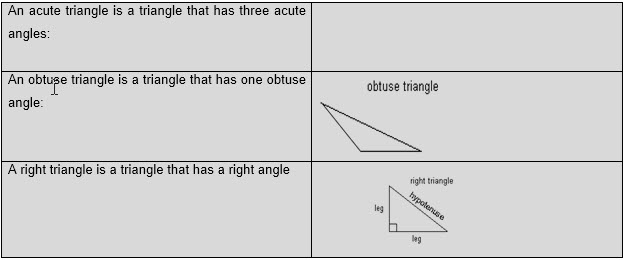The hypotenuse is the side opposite the right angle and the legs are the sides that meet at the right angle. The following three types of triangles are categorized by their sides: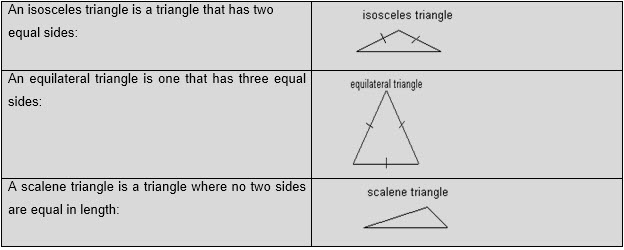Quadrilaterals are polygons with four sides. Table 4.1 contains examples of different types of quadrilaterals.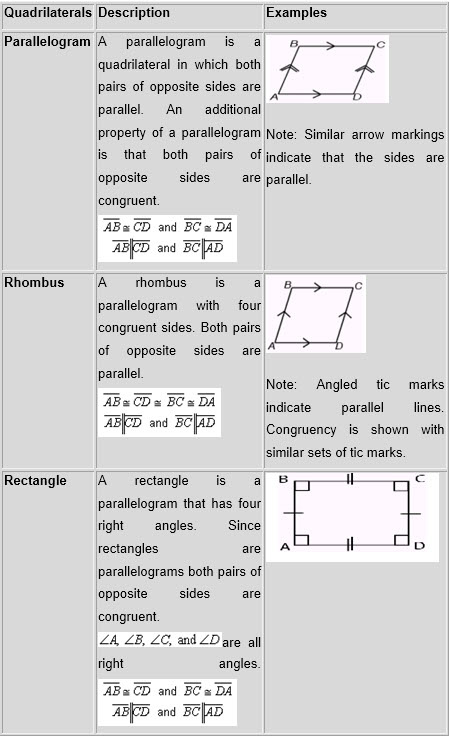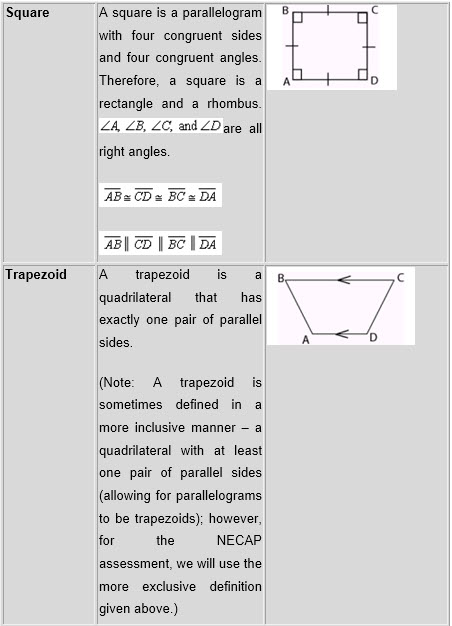#### 2.1.4 CirclesA circle is formed when a continuous line (circumference) starts at 0_ and ends at 360. It is a set of points that are equidistant from a fixed point called the centre. The radius (r on the diagram below) is the distance from the centre of the circle to any point on the circle and can be shown as a line segment connecting the centre to a point on the circle.

The diameter is a line segment that connects two points on the circle and goes through the centre of the circle.  It is always twice as long as the radius. A chord (line segment PQ on the diagram below) is any line segment whose endpoints are any two points on the circle. The circumference of a circle is the distance around the circle.An arc of a circle is the set of all points between and including two given points.One way to measure it is in degrees.  Keep in mind that the whole circle is 360 degrees. When naming an arc, it is best to use three points – the two endpoints and a point in between – versus just the two endpoints.  The reason is you can go clockwise or counter clockwise, which can make a difference when looking at the length of an arc. Arc ADC would start at point A and go clockwise through D and end at C.  Arc ADC is a 95 degree arc. Arc ABC would start at point A and go counter clockwise through B and end at C.  Since a circle is 360 degrees, then Arc ABC is a 360 – 95 = 265 degree arc. A line is tangent to a circle if it intersects the circle at exactly one point.The tangent line and the radius of the circle that has an endpoint at the point

of tangency are perpendicular to each other.

### 2.2 ANGLES

An angle measures the amount of turn. As the angle increases, the name changes

#### 2.2.1Right Angles

A right angle is an angle that is formed when 2 lines intersect at 90°.

#### 2.2.2The Acute Angle

An acute angle is any angle smaller than 90° i.e. smaller than a right angle.

#### 2.2.3 The Straight Angle

The straight angle is an angle that equals 180. Any straight line is 180 and is therefore a straight angle.

#### 2.2.4 The Obtuse Angle

Obtuse angles are any angles greater than 90° but smaller than 180° i.e. it is a rotation between a right angle and a straight angle.

#### 2.2.5 The Revolution

A revolution is any angle that is 360°. (I.e. 2 straight angles or 4 right angles)

#### 2.2.6 The Reflex Angle

A reflex angle is any angle greater than 180° but less than 360° i.e. it is an angle between a straight angle and a revolution.#### 2.2.7 Supplementary Angles

The sum of all the angles that lay along a straight line, will add up to 180°.These two angles (140° and 40°) are Supplementary Angles, because they add up to 180°.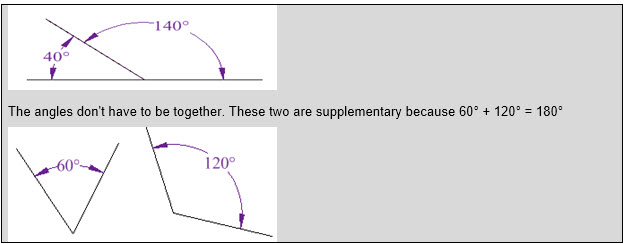#### 2.2.8 Complementary Angles

Two Angles are Complementary if they add up to 90 degrees (a Right Angle). These two angles (40° and 50°) are Complementary Angles, because they add up to 90°.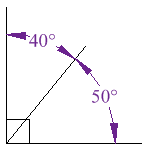#### 2.2.9 Opposite Angles

When two lines intersect each other, vertically opposite angles are equal:

#### 2.2.10 Parallel and transversal lines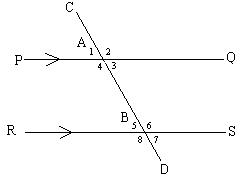Parallel

Parallel lines create many angles when a line intersects them. PQ//RS line PQ is parallel to RS (// means parallel to) and CD intersects lines PQ and RS. CD is called a transversal CD cuts PQ at A and RS at B to create many angles. Remember previous theorems:

1 = ∠3 and ∠2 = ∠4 (pairs of opposite angles on vertex A)

5 = ∠7 and ∠6 = ∠8 (pairs of opposite angles on vertex B)

∠1 + ∠2 = 180°; ∠3 + ∠4 = 180°; ∠1 + ∠4 = 180°; and ∠2 + ∠3 = 180° (angles along a line on line PQ at vertex A)

5 + ∠6 =180°; ∠7 + ∠8 = 180°; ∠5 + ∠8 = 180° and ∠6 + ∠7 = 180°(angles along a line on line RS at vertex B)

The pairs of angles created by the transversal on the parallel lines can be named as follows:

#### 2.2.11 Alternate Angles

4 and 6; 3 and 5; are called Alternate angles.

The alternate angles are equal to each other in pairs, i.e. 3 = 5 and 4 = 6.

Alternate angles are always inside parallel lines.

In conclusion basic geometry involves many terms which needs to be known in definition and also in application:

#### 2.2.12 Perpendicular Angles

When two lines meet each other at 90° then they are perpendicular to each other.

AB meets CD at 90°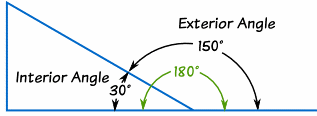#### 2.2.13 Interior Angle

An Interior Angle is an angle inside a shape.

#### 2.2.14 Interior Angle

An Interior Angle is an angle inside a shape.

### Exercise 1:

Go to exercise 1 in the sidebar. Complete it before going to next part of this course.

### 2.3 ESTIMATING, MEASURING AND CALCULATING

Estimation allows us to arrive at a ‘nearly correct’ answer that is close enough for all

practical purposes. Estimation is used when an exact answer is not yet required.

• When we want to estimate the size of a room, we will not necessarily measure it with a measuring tape, but give large steps that are roughly equal to a meter. In this case we are ‘estimating’ the size of the room.
• However, when we need to work out the exact answer, then we will measure the exact space with a suitable measuring instrument. This way we can establish an exact answer.
• We want to lay a carpet in a specific room. We will use a measuring tape to get the exact measurement. Otherwise, the carpet may be too small for the room or even too big.
• No matter whether you have estimated or measured, if you want to work out, for instance, the size of a room, you will need to calculate to arrive at a suitable answer.Length is always the longer side(s) of a shape, while breadth is the shorter sides of a shape. In the example below, length is indicated by the double line while breadth is indicated by the single line. Length and breadth is measured in meters. Any shape that has length and breadth is a two dimensional shape. You can estimate the length and breadth with your fingers. The length of one side is about six fingers long (i.e. one finger may be _2cm wide, so we can estimate that the length of this is 6 x 2 = 12cm) and the breadth is about 2 fingers long i.e. 2 x 2 =4cm.  Eventually you will be able to estimate the lengths by merely measuring it with your eye.

#### 2.3.2 Perimeter and Circumference

Perimeter

Perimeter is the distances from one point on the outside border of a shape, all the way around, back to the same point again. Perimeter and circumference is measured in meters.

To calculate the perimeter of a rectangle, square, parallelogram:

P = 2 length + 2 breadths and the answer is in mm, cm, m or km

To calculate the perimeter of any straight lined shape with more than four angles:

P = total sum of the length of all straight sides.

#### 2.3.3 Circumference

Circumference is similar to perimeter, but circumference is the word we use to describe the ‘perimeter’ of a circular shape.

To calculate the circumference of a circle:

C = 2πr (Where π is 22/7 or 3, 14)

#### 2.3.4 Area

Area is the amount of space a shape takes up in two dimensions i.e. length and breadth. If you measure a space such as a room, then you will estimate the length an breadth by using a stride. A stride is a very large step and is the distance between the heel of the back foot and the toe of the front foot. Sometimes the area that we have to calculate has an irregular shape. It seems impossible to then calculate the area, but a little clever thinking will help you to identify regular shapes within the irregular shape and you can then apply the regular formula.

a. Rectangle

To calculate the area of any rectangular shape we use the formula: A = lb (length x breadth) and the answer is in mm2 or cm 2 or m2 or km2

b. Circle

To calculate the area of a circle: A = πr2

c. Triangle

To calculate the area of a triangle:

A = 1/2base x height

d. Trapezium

There are two methods to determine the area of a trapezium. One method is by formula and the second method is by breaking the shape into rectangles and triangles.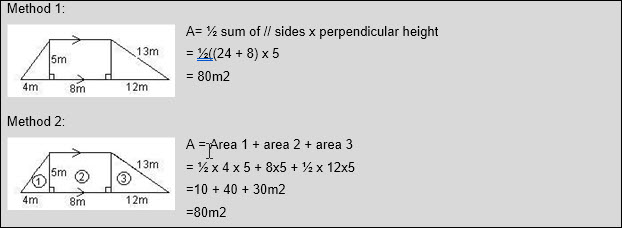#### 2.3.5 Volume

Volume is the space that a container can take on the inside. In order to determine the volume of a container, we need to add another dimension to the shape i.e. the height or depth. Up to now, we have calculated the area of a shape, working with the length and the breadth. The length is one dimension; the breadth is the second dimension. If we now add height or depth to a shape, we add a third dimension and we can see how much go into it.

#### 2.3.6 Angles

Where two lines, that are not parallel, intersect, and angle is formed. We measure angles in degrees (°) and we use a protractor to measure angles. All angles on the lines intersecting below are indicated by a dot.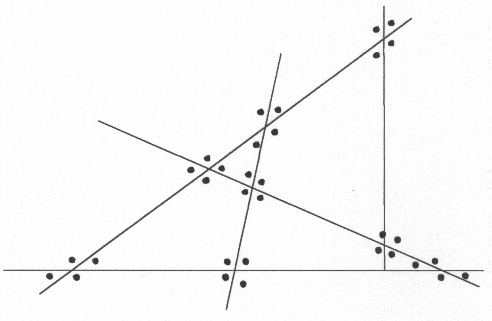We use a protractor as follows:

Place the 0° line of the protractor on top of the base line of the angle (in this case ABD).

Make sure the centre point of the protractor lies directly on top of where the two lines meet (point B). Start from the 0° point and move clockwise to where the secondary line cuts through the point on the protractor (in this case 55°).

If you want to measure angle CBD, your baseline would now be BC and you would place the 0° line of the protractor on top of BC, making sure the central point of the protractor is on top of point B and you will take the measurement(∠CBD will be 110° in this case).There are a number of common angles that we need to familiarize ourselves with. For information on these angles go back to section 1.4

### 2.4 DRAWING SCALES

Drawing scales are used to reduce the size of a large article so that it can be represented on a piece of paper. Maps normally use scale to indicate to which extent a piece of land has been reduced. The same principle is used in engineering drawings to illustrate the size of a component or engineering object.

A scale is the ratio of

• the distance between two points on a map vs.
• the actual distance between two points on a surface

Scales can be represented in one of three ways:

• as a ratio 1:1 500 000
• as a fraction 1/1 500 000

as a graphic scale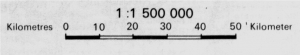Which means that for each 1 unit of measurement on the map, the distance is 1 500 000 of the same units of measurement on the real surface.

A scale drawing is a drawing where the accurate dimensions of an object or figure or area are reduced or enlarged. The scale drawing is the exact duplication of the original figure, shape or object, but it is smaller. An area is shown on a map as the drawing below. The scale on the map is

1:1 500 000. We measure the length and breadth of this drawing, which is 10cm x 5cm. We can then calculate the actual size of the area land, represented on the map.

1 : 1 500 000

1 x 5 : 1 500 000 x 5

5 : 7 500 000

So for every 5cm we measure on the drawing, the real measurement on land is

7 500 000cm (or 7,5 km).

1 : 1 500 000

1 x 10 : 1 500 000 x 10

10 : 15 000 000

The length in this case will be 15km on land.

Scales are very convenient in representing large areas on small scale so that an overall picture can be seen. The triangle below shows how scale minimizes and maximizes the size of the triangle.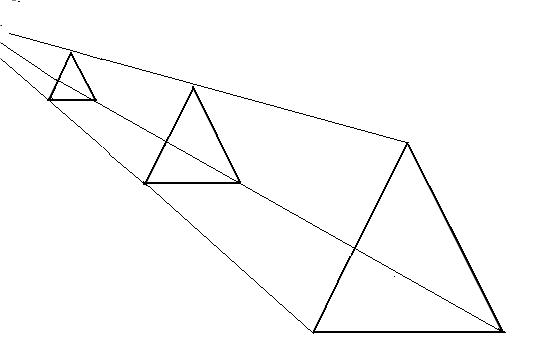### 2.5 TRANSFORMATIONS OF 2D GEOMETRIC FIGURES

A two-dimensional figure is a figure that has only two dimensions i.e. length and breadth.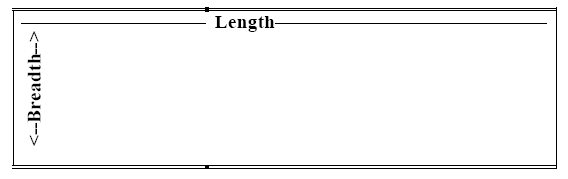We can change a two-dimensional figure, by adding to it height or depth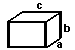A three-dimensional figure is a figure that has three dimensions i.e. length, breadth and height or depth.

#### 2.5.1 Symmetry

When a shape is symmetrical, it means that if we cut through the centre of the shape, both sides of the shape will be exactly the same size and shape. If we were to draw this triangle on a piece of paper and fold the paper in half, then the shape will be identical on both sides of the folding line.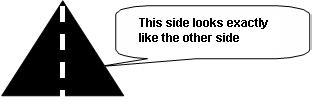Please note that should the line of symmetry be changed in the shape above, the shape will no longer be symmetrical.Here my dog “Flame” has her face made perfectly symmetrical with a bit of photo magic.

The white line down the center is the Line of Symmetry

#### 2.5.2 Perspective

Perspective is the point from where you are viewing an item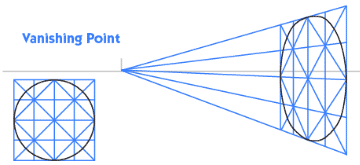### Exercise 2:

Go to exercise 2 in the sidebar. Complete it before going to the part of the course.

### Example 1

Three employees are hired to tar a rectangular parking lot of dimensions 20 m by 30 m. The first employee tars one piece and leaves the remaining shape, shown below, for the other 2 employees to tar equal shares. Show how they can share the job.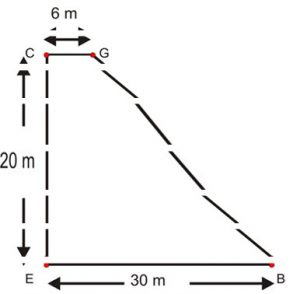• Draw a diagram
• Guess and check
• Use logic
• Use formulas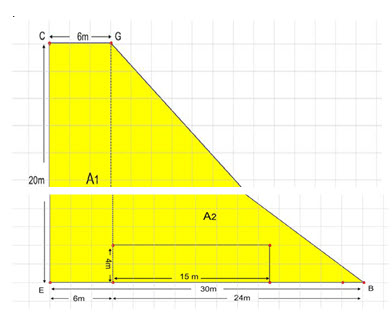A 1   = (b)(h)

= (6)( 20)

=120cm

A 2 =   bh/2

= (24)(20)

2

=240m

A2 has 120 m2 more the A1

∴take 60 m2 from A2 and add it to A1.

This can be rectangle of 15 × 4 taken from A2 (other configurations are possible).

The areas are now equal

A1 = 120 + 60 = 180 m2

A2 = 240 − 60 = 180 m

### Example 2

Find the area of this patch of pavement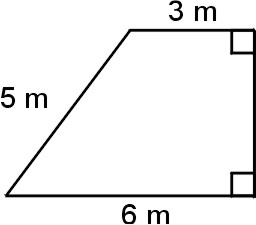Sample Solution

• Draw a diagram
• Make a scale model
• Use a formula
• Use technology (GSP®)
• Use tools (geoboard)

A=A 1 + A 2                                                                                        A= (a+b)(h)

=bh+ bh                                                                                   2

2

=3 (4)+3(4)                              OR                              =          (3+6)(4)

2                                                                                               2

=6+ 12

=18 m2                                                                        =          18m 2

With the given top and bottom lengths, you can use grid paper or a geoboard to determine a diagram that has a slanted side of 5 units, and thus determine the corresponding height. Once students know the height they can apply the area formula for a trapezoid; or separate the shape into a triangle and a rectangle and find each area and the sum of the areas.

### Example 3

The diagram is a square inside a circle and a square outside the circle.

Which has the greater area?

– the space between the circle and the inside square (Diagram A)? Or – the space between the circle and the outside square (Diagram B)?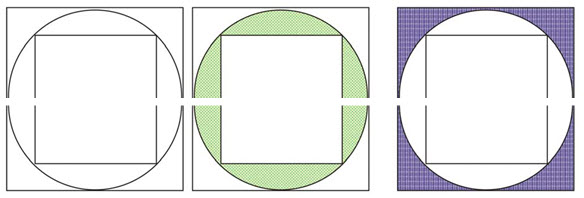This question may be easier to do if you rotate the inside square 45°.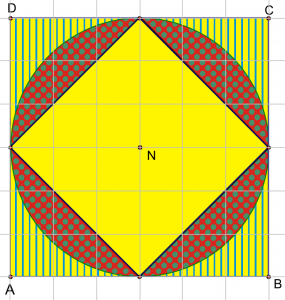You can cut out the areas in question and see how they “fit” together. This will provide an acceptable answer and justification, particularly if done with several-sized circles.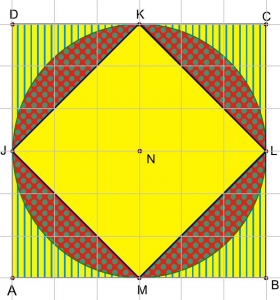Area DABC = 100.00 cm2

Area JMLK = 49.95 cm2

Area 􀀛NL = 78.22 cm2

(Area DABC) – (Area 􀀛NL) = 21.78 cm2

(Area 􀀛NL) – (Area JMLK) = 28.27 cm2

28.27 > 21.78

Therefore, the area between the circle and inside square is greater than the area between the circle and the outside square.

Note

NL is the GSP® symbol which refers to the area of the circle with radius NL.

### Exercise 3:

Go to exercise 3 in the sidebar. Complete it before going to next course.